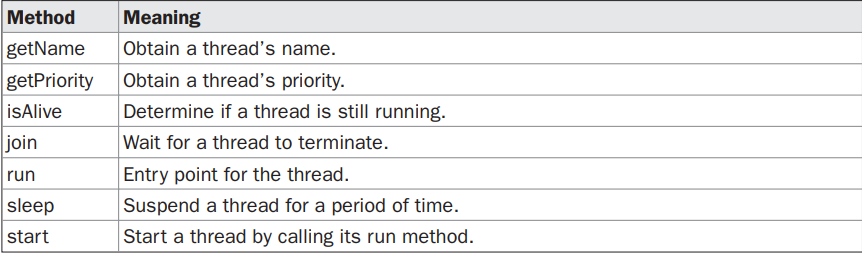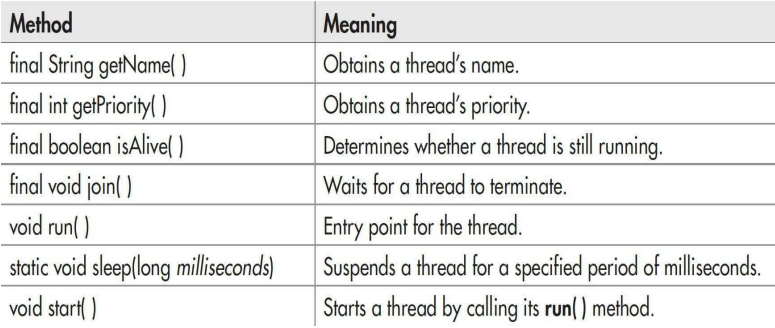In a thread-based multitasking environment, the thread is the smallest unit of dispatchable code. This means that a
a single program can perform two or more tasks at once.
By using multithreading, your program can execute another task during this idle time. For example, while one part of your program is sending a file over the Internet, another part can be reading keyboard input, and still another can be buffering the next block of data to send

• You create a thread by instantiating an object of type Thread.
• The Thread class encapsulates an object that is runnable. As mentioned, Java defines two ways -
• You can implement the Runnable interface.
• You can extend the Thread class.
In other words, To create a new thread, your program will either extend Thread or implement the Runnable interface.

• The first way to create a thread is to create a new class that extends Thread, and then to create an instance of that class.
• The extending class must override the run( ) method, which is the entry point for the new thread.
•  It must also call start( ) to begin the execution of the new thread. Here is the program rewritten to extend Thread -
Example -
 public class Main extends Thread {//main class   public void run() {     System.out.println("run() function runs a thread");   } }

 // online promotion house // web designing house public class Main implements Runnable { // Runnable interface    public void run() {     System.out.println("hello world");   } }

 public class Main extends Thread {   public static void main(String[] argument) {     Main thread = new Main();     thread.start();     System.out.println("This line is Not in thread");   }   public void run() { System.out.println("This is under run method hence inside thread"); int a = 4;   int b = 5;   a +=b;   System.out.println("The sum of 4, and 5 is = "+ a);   } }
Output -
 This code is outside of the thread The sum of 4, and 5 is = 9

2.Example of Implementing Thread(Runnable interface) -
 public class Main implements Runnable {   public static void main(String[] argument) {     Main object = new Main();     Thread thread = new Thread(object);     thread.start();     System.out.println("This line is Not in thread");   }   public void run() { System.out.println("This is under run method hence inside thread"); int a = 4;   int b = 5;   a +=b;   System.out.println("The sum of 4, and 5 is = "+ a);   } }
Output -
 This line is Not in thread This is under run method hence inside thread The sum of 4, and 5 is = 9

Methods used in Threading in JavaFew more methods -Prior to Java 2, a program used suspend( ), resume( ), and stop( ), which are methods defined by Thread, to pause, restart, and stop the execution of a thread. They have the following forms -
1.final void resume( )
2.final void suspend( )
3.final void stop( )